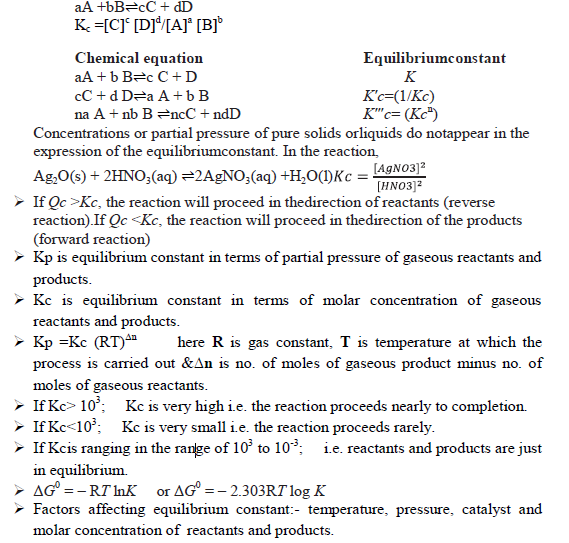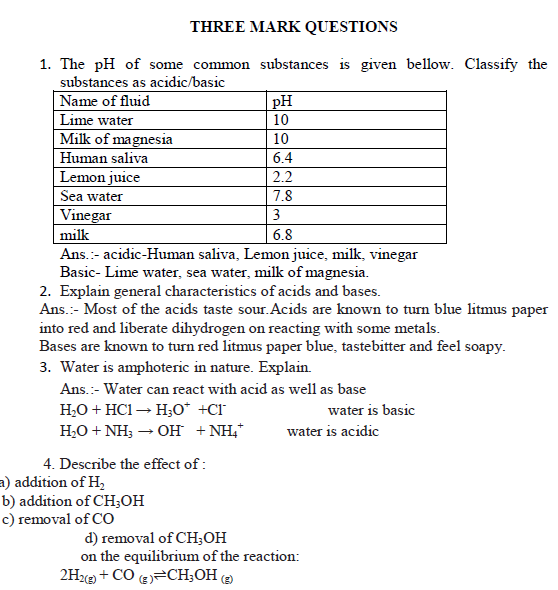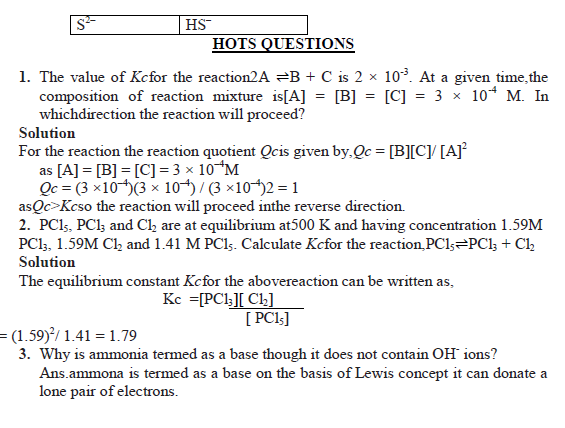# SCC Education

## EQUILIBRIUM notes with HOT Questions

EQUILIBRIUM
Equilibrium state- When rate of formation of a product in a process is in competition with rate of formation of reactants, the state is then named as “Equilibrium state” .
 Equilibrium in physical processes: solid ⇌ liquid ⇌ gas H2O(s )⇌ H2O(l) ⇌ H2O(vap)
Law of chemical equilibrium: At a given temperature, the product of concentrations of the reaction products raised to the respective stoichiometric coefficient in the balanced chemical equation divided by the product of concentrations of the reactants raised to their individual stoichiometric coefficients has a constant value. This is known as the Equilibrium Law or Law of Chemical EquilibriumLe Chatelier’s principle:- It states that a change in any of the factors that determine the equilibrium conditions of a system will cause the system to change in such a manner so as to reduce or to counteract the effect of the change.
 Arrhenius acids are the substances that ionize in water to form H+.
 Arrhenius bases are the substances that ionize in water to form OH-.
 Lewis acids are lone pair (of e-) accepters while Lewis bases are lone pair donators.
 Proton donor are acids while proton accepters are bases(Bronsted-Lowry concept).
 The acid-base pair thatdiffers only by one proton is called a conjugate acid-base pair. If Brönsted acid is a strong acid then its conjugate base is a weak base and vice versa.
Ionic product of water.Kw = [H+][OH–]
 pH = -log [H+] ; here[H+] is molar concentration of hydrogen ion.
 pH + pOH =14
 pKa + pKb =14
 Ka x Kb = Kw = ionic product of water=1 x 10-14
 Buffer solution :The solutions which resist change in pH on dilution or with the addition of small amounts of acid or alkali are called Buffer Solutions.
common ion effect: It can be defined as a shift in equilibrium on adding a substance that provides more of an ionic species already present in the dissociation equilibrium.
 Hydrolysis of Salts: process of interaction between water and cations/anions or both of salts is called hydrolysis.
The cations (e.g., Na+, K+,Ca2+, Ba2+, etc.) of strong bases and anions(e.g., Cl–, Br–, NO3–, ClO4– etc.) of strong acids simply get hydrated but do not hydrolyse, and therefore the solutions of salts formed from strong acids and bases are neutral i.e., their pH is 7.
 Salts of weak acid and strong base e.g.,CH3COONa are basic in nature.
 Salts of strong acid and weak base e.g.,NH4Cl, are acidic
 Salts of weak acid and weak base, e.g.,CH3COONH4. The pH is determined by the formula pH = 7 + ½ (pKa – pKb)
Solubility product- product of the molar concentrations of the ions in a saturated solution, each concentration term raised to the power equal to the no. of ions produced.

ONE MARK QUESTIONS
Q.1. Mention the factors that affect equilibrium constant.
Ans. Temperature, pressure, catalyst and molar concentration of reactants and products.
Q.2. What is ionic products of water?
Ans. Kw = [H+] [OH-]
Q.3. Write conjugate acids of H2O & NH3.
Ans. H3O+& NH4+.
Q.4. Define Arrhenius acids.
Ans. Arrhenius acids are the substances that ionize in water to form H+.
Q.5. Define the term degree of ionization.
Ans.Extent up to which an acid/base/salt ionize to form ions.
Q.6. What are Buffer solutions?
Ans.The solutions which resist change in pH on dilution or with the addition of small amounts of acid or alkali are called Buffer Solutions.
Q.7. Write Kc for the gaseous reaction- N2 + 3H2⇌ 2NH3
Ans. Kc=[NH3]2/[N2] [H2]3
Q.8. Out of H2O & H3O+ which is stronger acid?
Ans. H3O+.
Q.9. What is common ion effect?
Ans. Shift in equilibrium on adding a substance that provides more of an ionic species already present in the dissociation equilibrium.
Q.10. Write relationship between Kp and Kc for the gaseous reaction - N2 + O2 ⇌2NO
Ans. Kp = Kc asΔn is zero for the above said reaction.

TWO MARKS QUESTIONS

1. What is effect of catalyst on equilibrium constant „Kc‟?
Ans . A catalyst does not affect equilibrium constant because it speeds up both forward and backward reactions to the same extent.
2. State Le Chatelier‟r principle.
Ans.It states that a change in any of the factors that determine the equilibrium conditions of a system will cause the system to change in such a manner so as to reduce or to counteract the effect of the change.
3. What is meant by conjugate acid –base pairs? Explain.
Ans:
4. Classify the following bases as strong and weak bases: NaHCO3, NaOH, KOH, Ca(OH)2, Mg(OH)2.
Ans:-strong base NaOH, KOH ; weak bases NaHCO3,Ca(OH)2, Mg(OH)2.
5. The concentration of hydrogen ion in a sample of soft drink is 3.8 × 10–3M. What is its pH ?
Ans:-pH = – log[3.8 × 10–3] = – {log[3.8] + log[10–3]} = – {(0.58) + (– 3.0)} = – { – 2.42} = 2.42
Therefore, the pH of the soft drink is 2.42 and it is acidic.
6. The species: H2O, HCO3–, HSO4– and NH3 can act both as Bronsted acids and bases.For each case give the corresponding conjugate acid and conjugate base.
Ans :
7. Explain Lewis acids and bases with suitable examples.
Ans:-Lewis acids are lone pair (of e-) accepters while Lewis bases are lone pair donators. AlCl3 is a Lewis acid while NH3 is a Lewis base.
8. What is difference between alkali and bases? Give examples.
Ans:- An alkali is a water soluble base. All the alkalis are bases but all the bases are not alkali. Ex- NaOH is an alkali/base. Ca(OH)2 is a base but not an alkali.
9. Explain homogeneous and heterogeneous equilibrium giving examples.
Ans:- If all the reactants and products present in an equilibrium mixture are in same phase→homogeneous equilibrium. If all the reactants and products present in an equilibrium mixture are in different phase→ heterogeneous equilibrium. N2 (g) + 3H2(g) ⇌2NH3(g) homogeneous equilibrium CaCO3(s)⇌CaO(s) + CO2(g)heterogeneous equilibriumChemistry for class 11.....
elements-of-group-13-p-block-elements
states-of-matter-liquids-and-solids
geometric-isomerism-different-geometries
chemical-thermodynamics
introducation-of-carbon-chemistry
electrons-in-atom-and-periodic-table
hybridisation
intermolecular-forces-liquid-and-solids
niels-bohr-atomic-model
iupac-nomenclature-of-organic-compounds
chemical-bonding-molecular-geometry
molecular-orbital-theory
heisenberg-uncertainty-principle
some-basic-concepts-of-chemistry
equilibrium
environmental-chemistry
hydrogen
structure-of-atom
classification-of-elements